# Interesting Probability Examples Set 1

Go back to  'Probability'

The subject of probability gives rise to some very interesting questions, which we believe everyone should be exposed to, even if to a minor extent. In this section, we’ll do precisely that. The examples will clearly show you the depth of this subject.

Example – 23

A drunk mathematician called Pi  is performing a random walk along the number line as follows. His original position is x = 0. He takes a step forward or a step backward with equal probability. Thus, for example, if he is at x = 1, he can go to x = 2 or x = 0 with equal probability.

His home is at x = 3, while there is a pit at x = – 2. What is the probability that he’ll reach home before falling into the pit?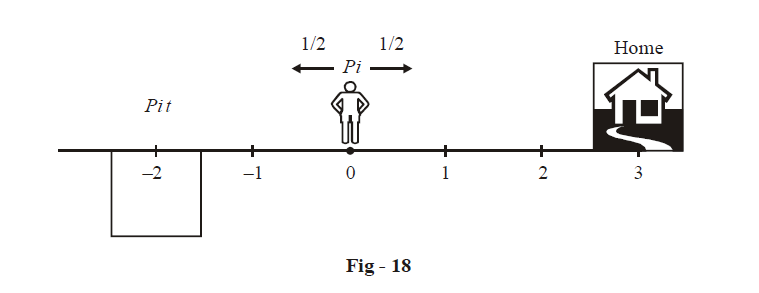Solution: Assume the required probability to be p. Thus, p denotes the probability P(E) where

E : Pi  reaches home before the pit

Now, let us draw the probability tree that will make E happen: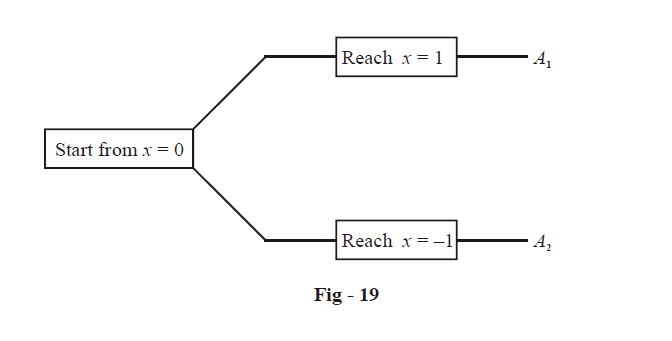What should the events A1 and A2 be and what should be their probabilities? Once Pi has reached x = 1, the situation is as follows: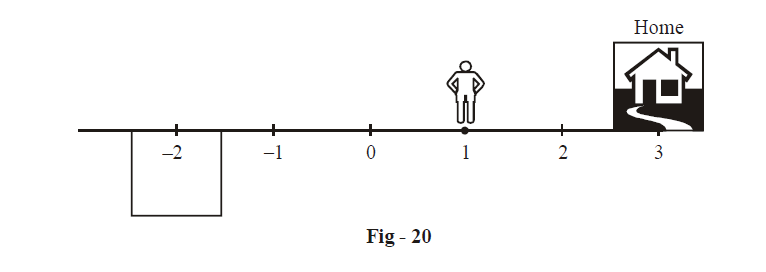A1 should therefore be the event that Pi reaches x = 3 before x = – 2. Now comes the crucial insight. Compare Fig-20 with Fig-18. The situations have been symmetrically reversed. In Fig -18, p was the probability that Pi reaches x = 3 before x = – 2, that is, Pi travels 3 units in one direction before travelling 2 in the other. In Fig-20, P(A1) is the probability that Pi travels 2 units in one direction before travelling 3 in the other, so we simply have

$P({{A}_{1}})=1-p$

Make sure you understand this argument carefully.

Coming to A2, Pi  has  reached x = – 1, he must return to x = 0, because he must not reach x = – 2. Once he is at x = 0, he comes back to the starting configuration, which means that now his probability of reaching x = 3 before x = – 2 is again p.

So let us complete the probability tree of Fig-19 now: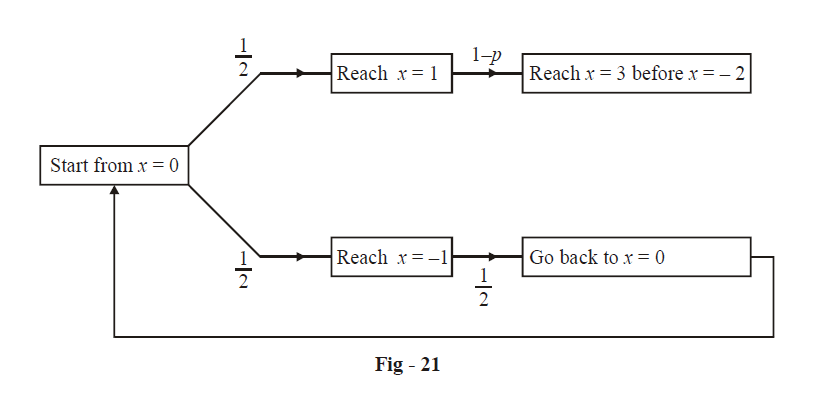Thus (pay attention to how we write this!),

$p\,\,=\,\,\frac{1}{2}\,\,\times \,\,\underset{\begin{smallmatrix} \text{Reaching}\ \ x=3\ \\ \text{ from}\ x=1 \end{smallmatrix}}{\mathop{(1-p)}}\,\,\,+\,\,\frac{1}{2}\,\,\,\times \,\,\,\frac{1}{2}\,\,\times \underset{\begin{smallmatrix} \text{Back}\ \text{to} \\ \,\,\,\,x=0 \end{smallmatrix}}{\mathop{p}}\,...\left( 1 \right)$

This relation is a form of recursion. In the definition (or expression) of p, we are using p again. Thus, recursion is, in plain words, the process a ‘procedure’ goes through when one of the steps of the ‘procedure’ involves rerunning the procedure. In this example, the ‘procedure’ of calculating p involved the step $$(x=0)\to (x=-1)\to (x=0),$$ at which point we have to rerun the ‘procedure’ of calculating p.

From (1),

\begin{align} &\quad\quad\;\; p=\frac{1}{2}-\frac{1}{2}p+\frac{1}{4}p \\ & \Rightarrow \quad \frac{5}{4}p=\frac{1}{2} \\ & \Rightarrow \quad p=\frac{2}{5} \\ \end{align}

Thus, there is a 40% chance that Pi will return home safely!

Recursions can also take the following form: suppose we have to calculate Tn, the nth term of some sequence, and suppose we are able to express Tn as some function of Tn – 1, the previous term:

${{T}_{n}}=f({{T}_{n-1}})$

This is also a recursion relation, for now we can use this relation repeatedly to descend, that is, to reach some Tm that is calculable:

${{T}_{n}}=f({{T}_{n-1}})=f(f({{T}_{n-2}}))=f(f(f({{T}_{n-3}})))=......=f(f(f(.....{{T}_{m}}))))....)$

For example, Tm   could be the first term of the sequence. Thus, here we are rerunning the ‘procedure’ f.

We’ll see an example of such a recursion in the solved examples.

Example – 24

After a fight, Adam and Eve decide to meet in Cafe Coffee Day to patch up. They fix up a 1 hour slot from 12:00 Noon to 1:00 PM, for this purpose. Each of them can reach CCD at any instant during this 1 hour time period.

However, still being angry with each other, each tells the other that he/she will wait at the most 10 minutes for the other person. If the other person does not turn up during these 10 minutes, he/she will go away.

What is the probability that Adam and Eve will meet?

Solution: The issue here is that there is nothing discrete that you can count. There are no discrete ‘favorable cases’ and no discrete ‘total cases’. This is because time as we perceive it is continuous; since each person can reach at any instant during the one hour slot, the arrival time of each is “ continuously distributed” across the one hour slot. This means, for example, that Adam can with equally likelihood arrive between, say 12 : 04 and 12 : 05 or between 12 : 48 and 12 : 49 etc. As another example, Eve can with equal likelihood arrive between, say 12 : 08 : 10 and 12 : 08 : 20 or between 12 : 59 : 49 and 12 : 59 : 59 etc.

Thus, if we divide the 1 hour slot into n sub-slots of the same size, each sub-slot can contain with equal likelihood Adam’s or Eve’s time of arrival. The problem is that there is no minimum size, for the sub-slots. You can have infinitesmally small sub-slots.

To counter this problem, we proceed as follows. Let x and y be Adam’s and Eve’s ‘normalised’ time of arrivals respectively, the word ‘normalised’ implying the following procedure:

If Adam arrives at 12 : 20, \begin{align}x=\frac{20}{60}=\frac{1}{3}\end{align}

If Eve arrives at 12 : 34,  \begin{align}y=\frac{34}{60}=\frac{17}{30}\end{align}

If Adam arrives at 12 : 29 : 08, \begin{align}x=\frac{29\frac{8}{60}}{60}\end{align}

:

:

and so on

Thus, normalised time means

$\left\{ \frac{\text{time}\ \text{of}\ \text{arrival}\ \text{since}\ 12:00}{1\ \text{hour}} \right\}$

It is clear that both x, $$y\in [0,\ 1]$$ . Now x and y are ‘uniformly distributed’ on [0, 1], meaning that both x and y can lie anywhere in [0, 1] with equal likelihood. More precisely stated, if we select an interval of length $$\Delta$$ anywhere in [0, 1], x and y have equal probability (respectively) of lying in any such interval.

Thus, the possible (continuous) sample for x and y is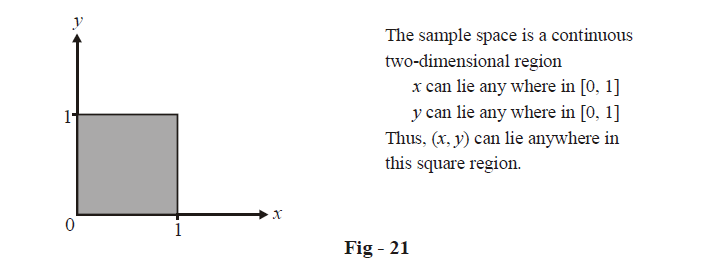This figure gives us the ‘total’ region corresponding to x and y. Now let us find out the ‘favorable’ region: Adam and Eve will meet only if their arrival times are separated by at the most 10 minutes, that is, only if

$|x-y|\ \le \frac{1}{6}\,\,\,\,\left\{ \text{If you normalise 10 minutes, you get $$\frac{1}{6}$$} \right\}$

Let us now plot this region within the shaded square of Fig-21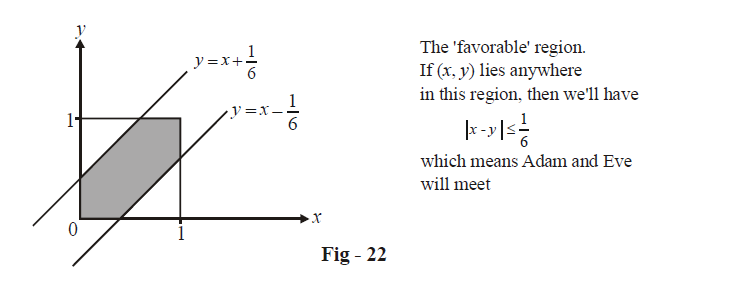The area of this region is \begin{align}\frac{11}{36}\end{align} (verify)

Thus, the required probability p is

\begin{align}& \;\;p=\frac{\text{Area}\ \text{of}\ \text{Favorable}\ \text{Region}}{\text{Area}\ \text{of}\ \text{Total}\ \text{Region}} \\&\quad =\frac{11/36}{1} \\ & \quad=\frac{11}{36} \\ \end{align}

Note how in this continuous distribution, we have “area of” instead of “number of”.

To conclude, there is a  \begin{align}\frac{11}{36}\end{align} or 30.55% chance that Adam and Eve will meet (and hopefully patch-up!).